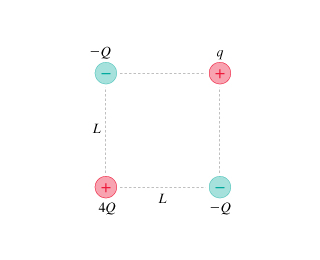# I need some help with mastering physics2?

+1 vote

edited

## I need some help with mastering physics2?

The figure shows four charges at the corners of a square of side L. Assume q and Q are positive.What is the magnitude of the net force on q?

(Give your answer in terms of Q, q, L and constant k.)

F = ______________

thanks

by
selected by

The formula for electrostatic attraction/repulsion is

F = k*q1*q2/r^2

where q1 = q, q2 = Q, and r = L. The direction is toward or away from the Q being considered.

Individually write expressions for the forces on q due to the charges at the other three corners. Then form the resultant of those 3 forces. (Make use of symmetry while forming the resultant.)

Lorem ipsum dolor sit amet, consectetur adipiscing elit, sed do eiusmod tempor incididunt ut labore et dolore magna aliqua. Ut enim ad minim veniam, quis nostrud exercitation ullamco laboris nisi ut aliquip ex ea commodo consequat. Duis aute irure dolor in reprehenderit in voluptate velit esse cillum dolore eu fugiat.
by

Start by drawing force vectors on charge q. You will notice that if the force vectors from both of the -Q charges are combined, the resultant will line up with the force vector from the +4Q charge.

So, use pythagorean theorem to find the resultant of the two -Q forces using Coulomb's equation F = k*q1*q2/r^2, and then use this result to subtract from the force of the +4Q charge.

The final answer will look similar this:

F = (K|4Q∣∣q∣ / 2L^2) − (sqrt(2)*K|Q∣∣q∣∣ / L^2)

Lorem ipsum dolor sit amet, consectetur adipiscing elit, sed do eiusmod tempor incididunt ut labore et dolore magna aliqua. Ut enim ad minim veniam, quis nostrud exercitation ullamco laboris nisi ut aliquip ex ea commodo consequat. Duis aute irure dolor in reprehenderit in voluptate velit esse cillum dolore eu fugiat.